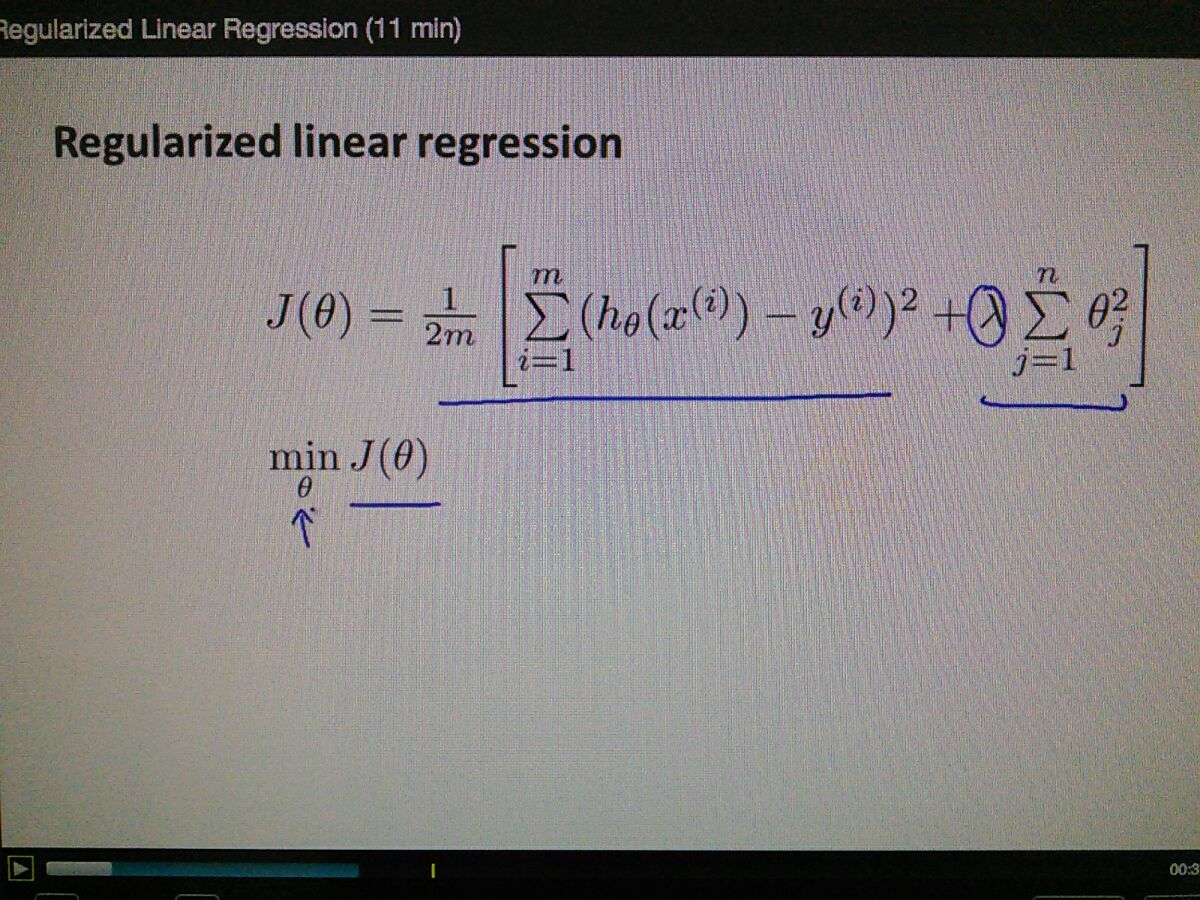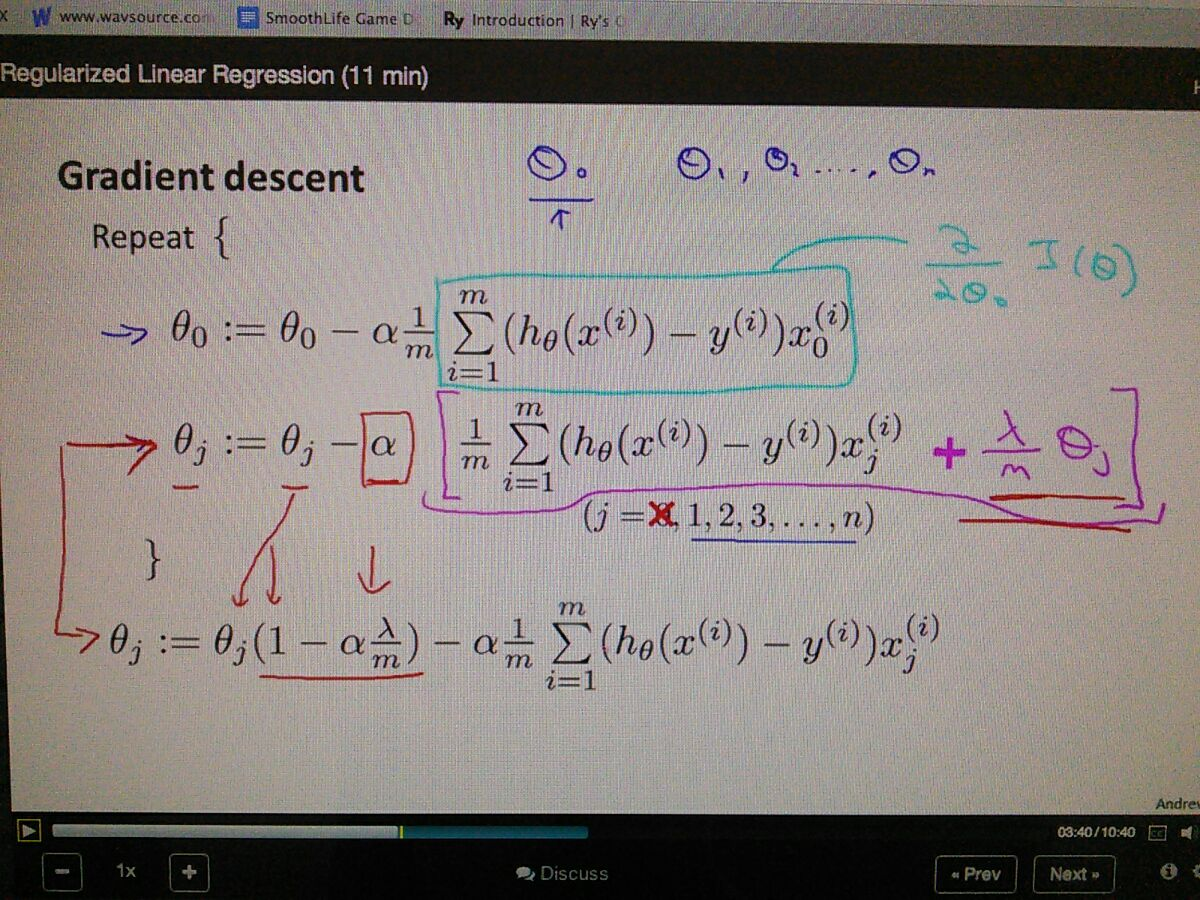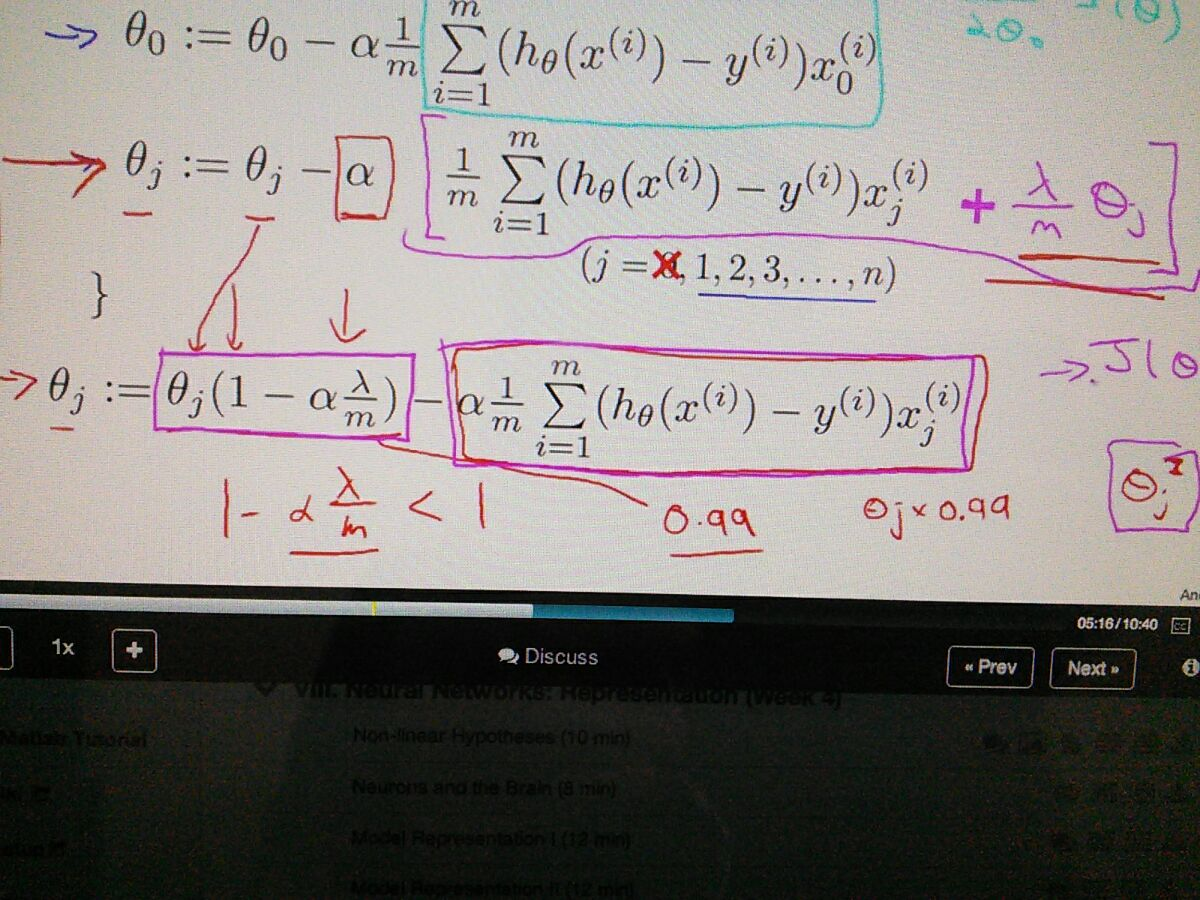• regularized both gradient descent and normal equation algorithms for linear regressioncalculate min theta for minimizing J thetaRegularization penalize all thetas except theta zero

so the box purple is the regularized derrivative for J theta
and cyan box is derrivative J theta without Regularization

then the separated both zero(?)? and the rest function become one line that shown at the bottomm often high because of many training set making it really small,  in addition becoming quadratic

next for normal equation....